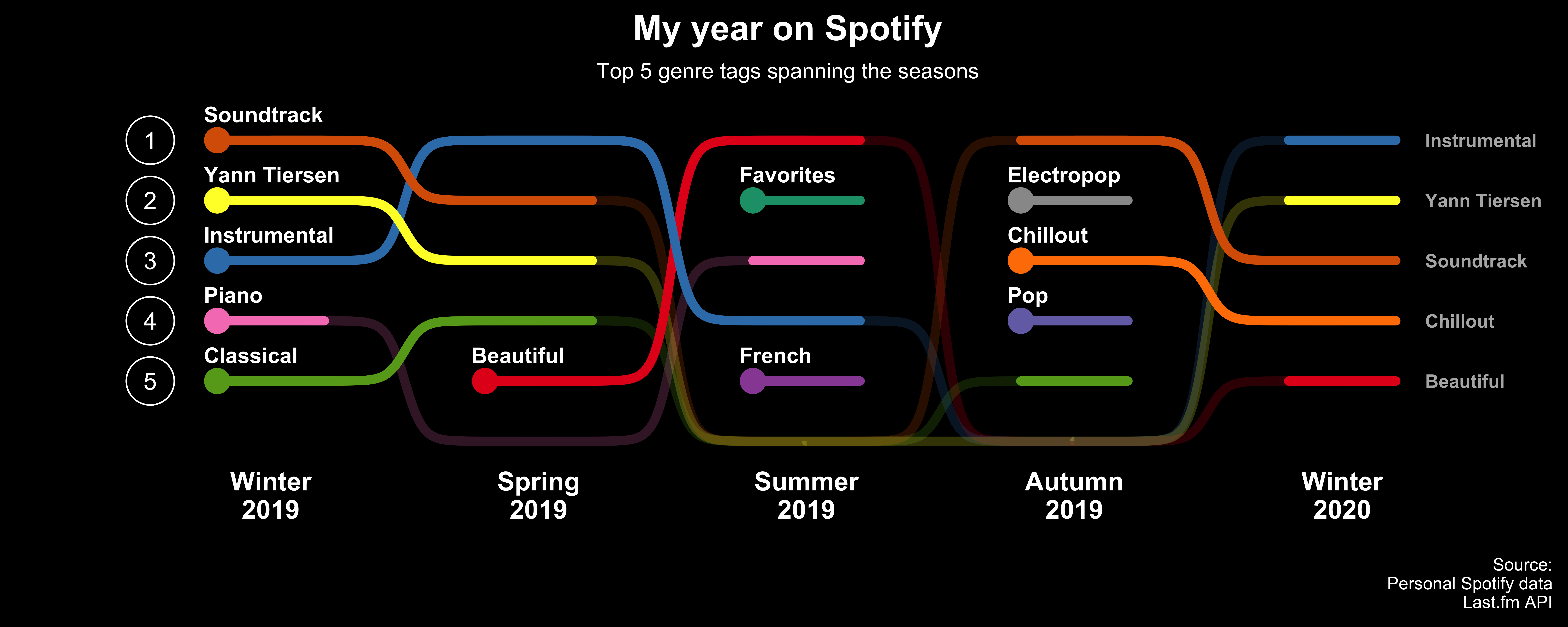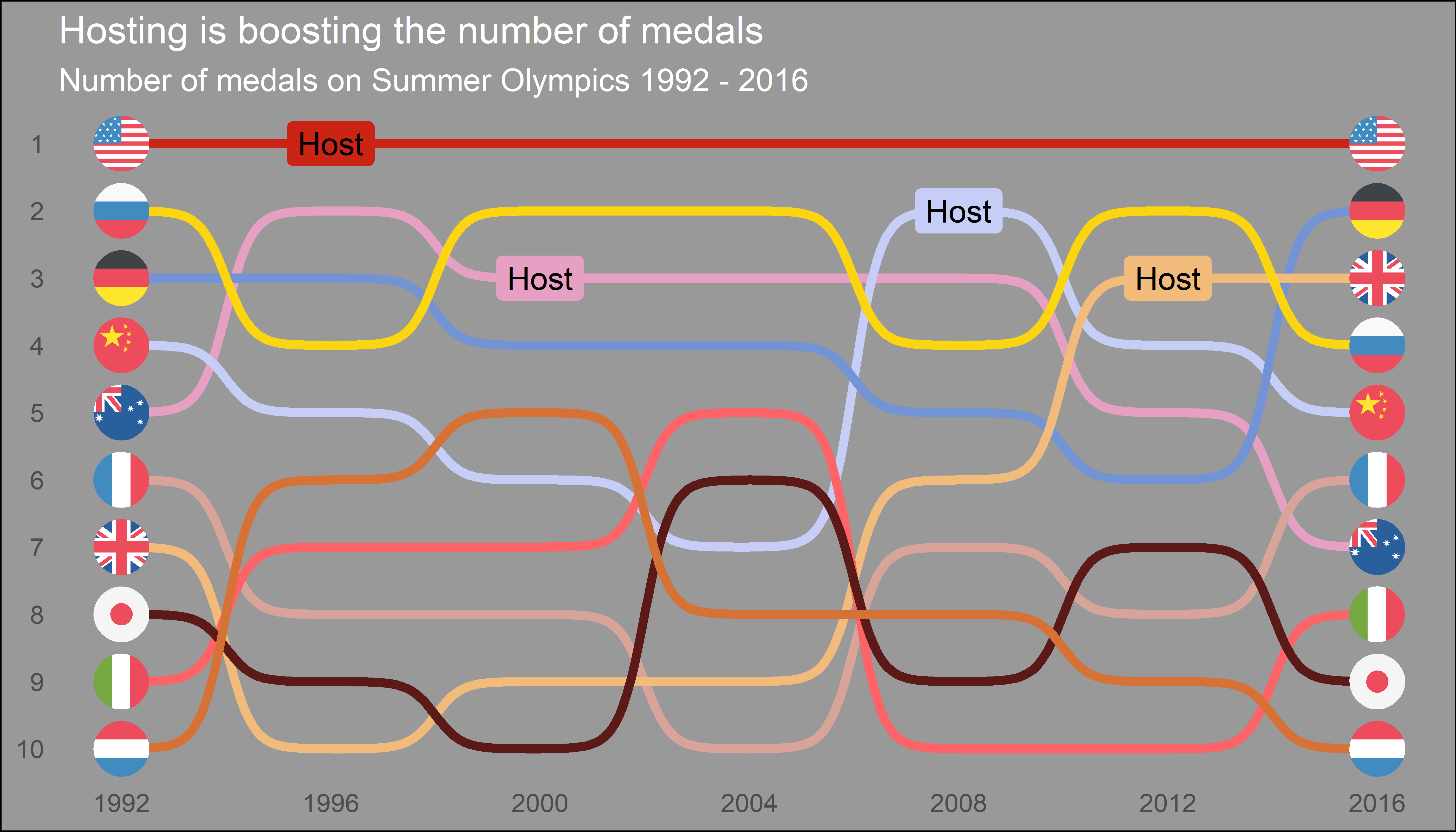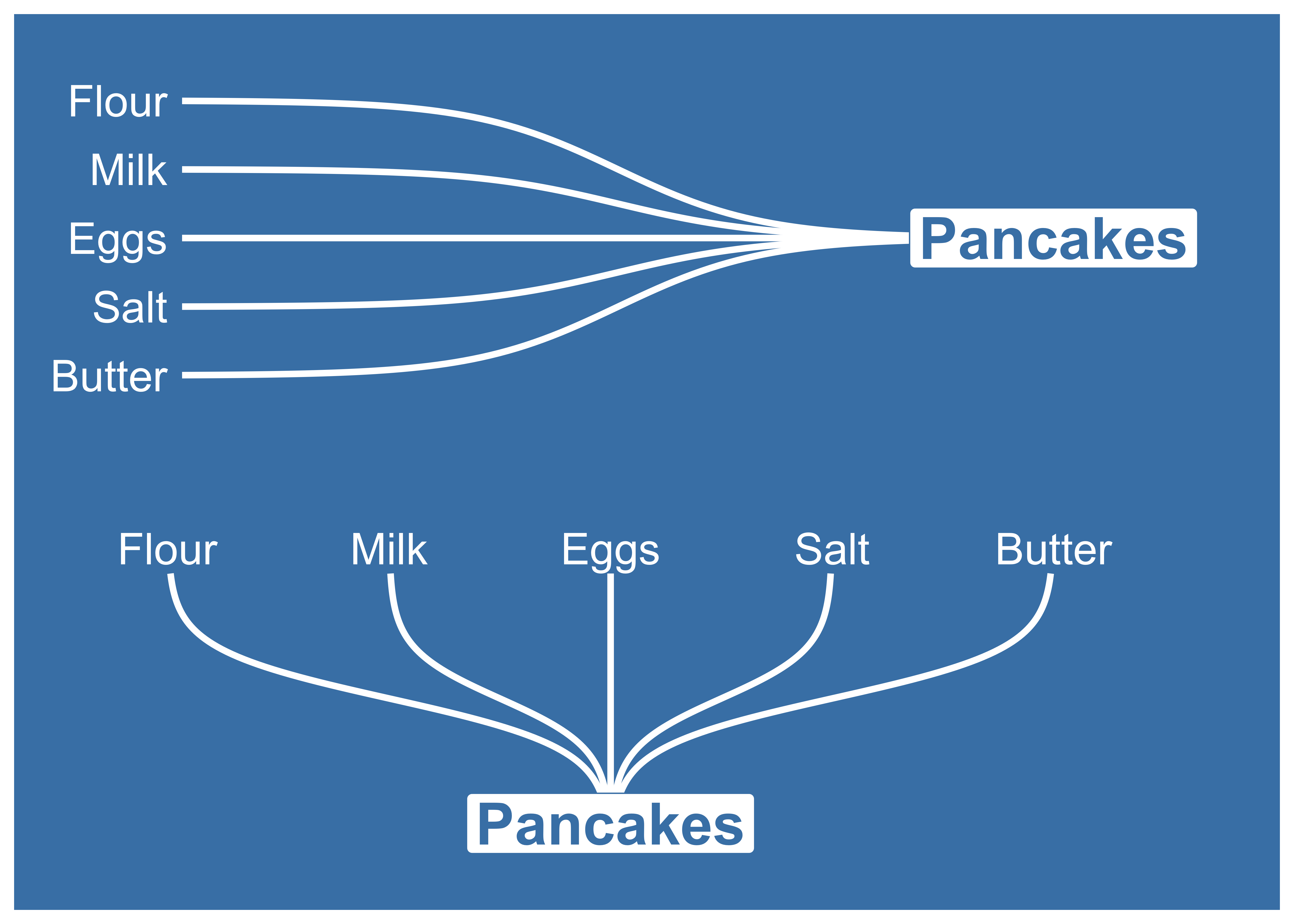Need help with ggbump?
Click the “chat” button below for chat support from the developer who created it, or find similar developers for support.davidsjoberg384 Stars25 ForksOther16 Commits5 Opened issuesDescription

A geom for ggplot to create bump plots!
?

Need anything else?ggbumpThe R package

ggbump
creates elegant bump charts in ggplot. Bump charts are good to use to plot ranking over time, or other examples when the path between two nodes have no statistical significance. Also includes functions to create custom smooth lines called sigmoid curves.

Installation

You can install ggbump from CRAN with:

install.packages("ggbump")

Or the latest development version from github with:

devtools::install_github("davidsjoberg/ggbump")

Bump chart examples

Basic example:Flags could be used instead of names:Sigmoid curves examples

With

geom_sigmoid
you can make custom sigmoid curves:With

geom_sigmoid
you have the flexibility to make more complex plots:Tutorial

Prep

Load packages and get some data with rank:

if(!require(pacman)) install.packages("pacman")
library(ggbump)

df

| country | year | value | | :------ | ---: | ----: | | India | 2011 | 492 | | India | 2012 | 246 | | India | 2013 | 246 | | Sweden | 2011 | 369 | | Sweden | 2012 | 123 | | Sweden | 2013 | 492 |

To create a ranking column we use

rank
from base R. We specify
ties.method = "random"
to make sure that each country have different rankings if they have the same value.
df %
group_by(year) %>%
mutate(rank = rank(value, ties.method = "random")) %>%
ungroup()

| country | year | value | rank | | :------ | ---: | ----: | ---: | | India | 2011 | 492 | 4 | | India | 2012 | 246 | 2 | | India | 2013 | 246 | 2 | | Sweden | 2011 | 369 | 3 | | Sweden | 2012 | 123 | 1 | | Sweden | 2013 | 492 | 4 |

Make a bump chart

Most simple use case:

ggplot(df, aes(year, rank, color = country)) +
geom_bump()Pimp the bump chart!

Improve the bump chart by adding:

• A point for each rank observation.
• Choose a minimal theme, I use
theme_minimal_grid()
from
cowplot
.
• Choose nice colors so it does not look generic ggplot. I use a palette from
wesanderson
.
• Remove legend and add labels at the start and end of the bumpy ride.
• Reverse the y-axis to get rank 1 at the top.
• Adjust the ‘smoothness’ of the lines by setting
smooth
to 8. Higher means less smooth.
ggplot(df, aes(year, rank, color = country)) +
geom_point(size = 7) +
geom_text(data = df %>% filter(year == min(year)),
aes(x = year - .1, label = country), size = 5, hjust = 1) +
geom_text(data = df %>% filter(year == max(year)),
aes(x = year + .1, label = country), size = 5, hjust = 0) +
geom_bump(size = 2, smooth = 8) +
scale_x_continuous(limits = c(2010.6, 2013.4),
breaks = seq(2011, 2013, 1)) +
theme_minimal_grid(font_size = 14, line_size = 0) +
theme(legend.position = "none",
panel.grid.major = element_blank()) +
labs(y = "RANK",
x = NULL) +
scale_y_reverse() +
scale_color_manual(values = wes_palette(n = 4, name = "GrandBudapest1"))geom_bump with factors (development version only)

You can use

geom_bump
with factors or character as x axis. Just remember to keep an eye on factor order.
# Original df
df %
mutate(season = factor(season, levels = unique(season)))

Add manual axis labels to plot

ggplot(df, aes(season, rank, color = player)) + geom_bump(size = 2, smooth = 20, show.legend = F) + geom_point(size = 5, aes(shape = player)) + theme_minimal_grid(font_size = 10, line_size = 0) + theme(panel.grid.major = element_blank(), axis.ticks = element_blank()) + scale_color_manual(values = wes_palette(n = 4, name = "IsleofDogs1"))Feedback

If you find any error or have suggestions for improvements you are more than welcome to contact me :)